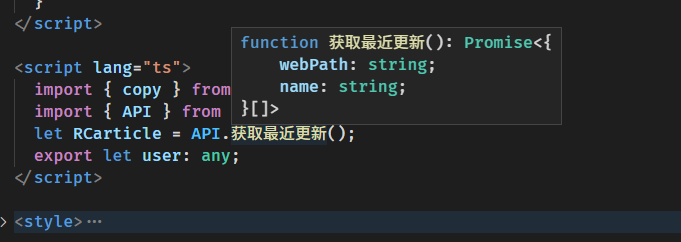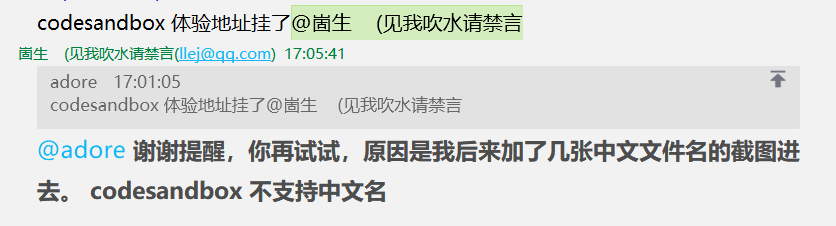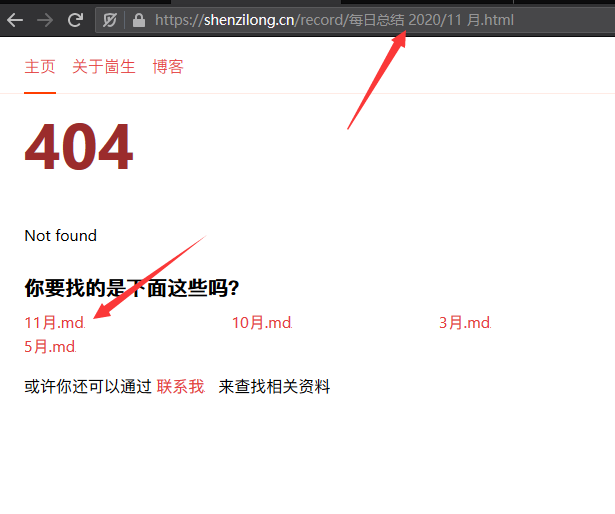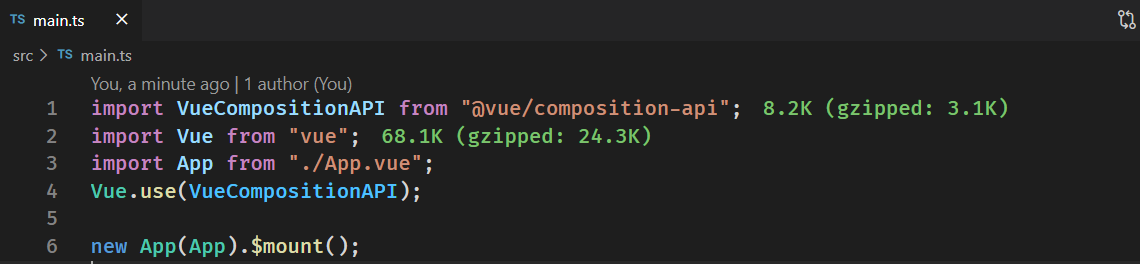# 2020/11 每日总结### 我的博客改为使用思源还有的一点小缺陷• 引用其他块的渲染还没写好（2020/11/8 号解决了）

• 还有嵌入内容块的渲染需要实现

## 加速度传感器与角速度传感器（陀螺仪）导致的窃听漏洞lute 通过 lute.SetHeadingAnchor(true); 可以配置渲染 h 系列的标签的时候加入一个 a 标签链接到该标题 效果如图## 一个简简单单的 TypeScript RPC 解决方案xml:base="https://shenzilong.cn/关于/赞助.html" 这样的属性可以设置 XML 的 base 路径，今天给我的 feed 加上了这样的路径 ，但不知道是不是我使用的 rss 阅读器的问题，他好像还是没有办法正确识别。

codesandbox 体验地址 codesandbox 的类型提示还不太行，本地开发是没有问题的

demo github 地址#### 0x00 服务端方法// apis/time.ts
export function currentTime() {
return Date.now();
}

export function currentTime2(toLocaleString: boolean) {
if (toLocaleString) {
return new Date().toLocaleString();
} else {
return Date.now();
}
}


#### 0x01 聚合// apis/index.ts
export * from "./time";


#### 0x02 Remote Procedure Call !Remote Procedure Call 要说的高大上呢那也有很多可以做的细节，但我们追求简简单单。

// router/rpc.ts
import * as apis from "../apis";

export async function post(req: any, res: any) {
const data = [] as any[];
req.on("data", function (chunk) {
data.push(chunk);
});
req.on("end", async () => {
const { method, data: _data } = JSON.parse(data.join(""));
const result = await apis[method](..._data);
"Content-Type": "application/json"
});
res.end(JSON.stringify(result));
});
}


#### 0x03 TypeScript ! 🎉//  rpc.ts
/** ═════════🏳‍🌈 超轻量级的远程调用，完备的类型提示！ 🏳‍🌈═════════  */
import type * as apis from "./apis";
type apis = typeof apis;
type method = keyof apis;

/** Remote call ， 会就近的选择是远程调用还是使用本地函数 */
export function RC<K extends method>(
method: K,
data: Parameters<apis[K]>
): Promise<unPromise<ReturnType<apis[K]>>> {
if (typeof window !== "undefined") {
// 客户端运行
return fetch("/rpc", {
method: "POST",
body: JSON.stringify({ method, data }),
"content-type": "application/json"
}
}).then((r) => r.json());
} else {
// 服务端运行，使用 import 的原因是避免 apis 的代码被打包发送到客户端
return import("./apis/index").then(async (r: any) => {
return await r[method](...data);
});
}
}

/** 解开 promise 类型包装 */
declare type unPromise<T> = T extends Promise<infer R> ? R : T;

// 示例 1 直接使用 RC

RC("currentTime", []).then((r) => console.log("服务器当前时间", r));
RC("currentTime2", [true]).then((r) => console.log("服务器当前时间本地化", r));

/** 包装了一次的 RC 方便跳转到函数定义  */
export const API = new Proxy(
{},
{
get(target, p: method) {
return (...arg: any) => RC(p, arg);
}
}
) as apisPromiseify;

/** apis 中包含的方法可能不是返回 promise 的，但 RC 调用后的一定是返回 promsie */
type apisPromiseify = {
readonly [K in keyof apis]: (
...arg: Parameters<apis[K]>
) => Promise<unPromise<ReturnType<apis[K]>>>;
};

// 示例 2 通过 API 对象调用对应方法，这里的优点是可以直接跳转到对应函数的源码处

API.currentTime().then((r) => console.log("服务器当前时间", r));
API.currentTime2(true).then((r) => console.log("服务器当前时间本地化", r));



#### 总结## 2020-11-13### 数组求和/** 数组求和 */
export function ArraySum<T>(arr: T[], f: (el: T) => number) {
return arr.map(f).reduce((a, b) => a + b, 0);
}


### 数组去重 ＆ 求和/** 数组去重
* 1. 可以设置函数来决定什么样算重复
* 2. 可以设置函数来决定遇到重复的结果该怎么合并
*/
export function Array去重<T>(
arr: T[],
/** 需要返回该对象的一个唯一标识（需要是原始值 用于和其他的进行比较） */ f: (el: T) => unknown = (el: T) => el,
/** 用于决定和重复对象的合并方式，默认取后面的值，丢弃旧值 */ mergeF: (a: T, b: T) => T = (a, b) => b,
) {
let result = [] as T[];
let label = [] as unknown[];

for (const el of arr) {
const id = f(el);
const i = label.findIndex((el) => el === id);
if (i !== -1) {
result[i] = mergeF(result[i], el);
} else {
label.push(id);
result.push(el);
}
}

return result;
}



### 数组取随机 n 个元素/** 从数组中随机取 n 个元素 */
export function sampleSize<T>([...arr]: T[], n = 1) {
let m = arr.length;
while (m) {
const i = Math.floor(Math.random() * m--);
[arr[m], arr[i]] = [arr[i], arr[m]];
}
return arr.slice(0, n);
}


## 使用编辑距离算法为自己的博客生成 404 页面的导流推荐### 编辑距离算法/** 求两个数组的编辑距离 */
export function minDistance<T>(l1: T[], l2: T[], equal?: (i1: number, i2: number) => boolean): number {
if (equal === undefined) {
/** 比较对应位置的元素是否相等 */
return minDistance(l1, l2, (i1, i2) => l1[i1] === l2[i2]);
}
const len1 = l1.length;
const len2 = l2.length;

let matrix = [] as any;

for (let i = 0; i <= len1; i++) {
// 构造二维数组
matrix[i] = [];
for (let j = 0; j <= len2; j++) {
// 初始化
if (i == 0) {
matrix[i][j] = j;
} else if (j == 0) {
matrix[i][j] = i;
} else {
// 进行最小值分析
let cost = 0;
if (!equal(i - 1, j - 1)) {
// 相同为0，不同置1
cost = 1;
}
const temp = matrix[i - 1][j - 1] + cost;

matrix[i][j] = Math.min(matrix[i - 1][j] + 1, matrix[i][j - 1] + 1, temp);
}
}
}
return matrix[len1][len2]; //返回右下角的值
}



## #图/表情包# 鸽子漫步## #图/常规# 中华人民共和国国旗#分享/链滴/块查询#

#vue/composition-api# 获取当前实例的方法 ： getCurrentInstance() 尤其需要注意的是 ctx.root !== this 我一开始错误的以为 ctx.root 就等于选项式风格中方法的 this 了，但实际上不是的 (我也不清楚我为什么会有这么奇怪的认为 🤣，仔细想想 root 怎么会是 当前实例呢？)

（1）量入为出，适度消费
（2）避免盲从，理性消费
（3）保护环境，绿色消费
（4）勤俭节约，艰苦奋斗

## #图/常规# 唐僧五指山放悟空## uniapp 体积优化秘技#分享/dcloud/uniapp 体积优化秘技#### 原理uni-app 会将 main.js 中的代码 copy 一份到所有的 生成后的 js 文件中，这是没有必要的，我们将这些代码移动到其他文件然后被打包到 vendor.js 就可以避免这个问题。

#uniapp/坑# 在代码中 import("page.json") 是没有办法拿到内容的，GitHub 相关吐槽 (这里面有一个解决方案) ，我在里面提了一个使用 webpack 的 loader 来获取的方案

import("!!raw-loader!../pages.json").then((r) => {
console.log("[r]", r);
});


by 崮生 from 崮生 • 一些随笔 🎨,欢迎 赞助本文